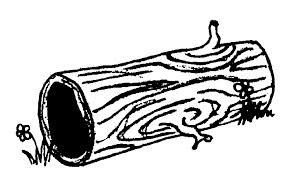# $xyz$

Algebra Level 1Given that $x, y$ and $z$ are positive real that are not 1, evaluate

$x^{log(\frac{y}{z})} \times y^{log(\frac{z}{x})} \times z^{log(\frac{x}{y})} .$

×